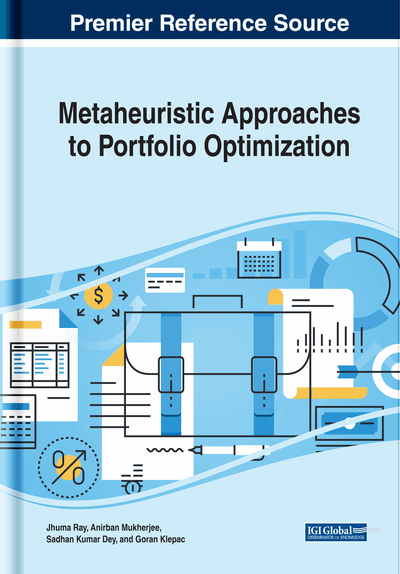# The Genetic Algorithm: An Application on Portfolio Optimization

Burcu Adıguzel Mercangöz (Istanbul University, Turkey) and Ergun Eroglu (Istanbul University, Turkey)
DOI: 10.4018/978-1-5225-8103-1.ch007

## Abstract

The portfolio optimization is an important research field of the financial sciences. In portfolio optimization problems, it is aimed to create portfolios by giving the best return at a certain risk level from the asset pool or by selecting assets that give the lowest risk at a certain level of return. The diversity of the portfolio gives opportunity to increase the return by minimizing the risk. As a powerful alternative to the mathematical models, heuristics is used widely to solve the portfolio optimization problems. The genetic algorithm (GA) is a technique that is inspired by the biological evolution. While this book considers the heuristics methods for the portfolio optimization problems, this chapter will give the implementing steps of the GA clearly and apply this method to a portfolio optimization problem in a basic example.
Chapter Preview
Top

## Evolutionary Algorithms

Evolutionary Algorithms (EAs) are the metaheuristics algorithms that are based on population (Vejandla, 2009). The algorithms use processes from the biological evolution such as selection, mutation and recombination (Slim et al. 2011). These algorithms commonly use the natural evolution concept. There are several heuristics classified as Evolutionary Algorithms (Streichert, 2002). The common characteristics of EA can be listed as;

• 1.

Easy to implement,

• 2.

Flexible,

• 3.

Quantitative,

• 4.

Allows wide variety of extensions and constraints different from traditional methods,

• 5.

Easily combined with other optimization techniques,

• 6.

Can be extended to Multi-objective optimization.

## Key Terms in this Chapter

Population: The set of solutions.

Markovitz Mean Variance Theory: The Financial theory that is suggested by Markowitz. According to the theory, the investors should select the optimum portfolios that are appropriate for them, at a certain expected level of return, from the portfolios with the minimum and maximum returns at a certain risk level.

Evolutionary Algorithms: Evolutionary algorithms are the population-based metaheuristic optimization algorithms that are inspired by biological evolution.

Offspring: Creating the next generation with new chromosomes.

Chromosome: Every row in the population matrix that represent the candidate solution of the problem.

Genetic Algorithm: A heuristics algorithm that is based on the mechanism of natural selection and natural genetics.

Sharpe Ratio: Sharpe ratio is used to measure the return of the portfolio according to risk.

Gene: Any cell in the chromosome.

## Complete Chapter List

Search this Book:
Reset